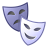# Create raster list by reading .tif files in multiple folders

2789
10
09-13-2017 11:13 AMNew Contributor III

Hi,

I need to read multiple folders and read the .tif files and hold it a rasterlist. I have mycode below but it holds only last year tif. files.

Can somebody help me to read all the .tif files and hold in the raster list. ?

``````years = [1981,1982,1983]
for year in years:
lstFiles = []
arcpy.env.workspace = r'H:\PRISM_800m_weekly_sum' + "\\" + str(year)
lstFiles = arcpy.ListRasters("*","TIF")
for raster in lstFiles:
print(raster)
lstFiles.sort()‍‍‍‍‍‍‍‍

>>> lstFiles
[u'Week_1_Sum1983_10.tif', u'Week_1_Sum1983_11.tif', u'Week_1_Sum1983_9.tif', u'Week_2_Sum1983_10.tif', u'Week_2_Sum1983_11.tif', u'Week_2_Sum1983_9.tif', u'Week_3_Sum1983_10.tif', u'Week_3_Sum1983_11.tif', u'Week_3_Sum1983_9.tif', u'Week_4_Sum1983_10.tif', u'Week_4_Sum1983_11.tif', u'Week_4_Sum1983_9.tif', u'Week_5_Sum1983_10.tif', u'Week_5_Sum1983_11.tif', u'Week_5_Sum1983_9.tif']
‍‍‍‍‍‍‍‍‍‍‍‍‍‍‍‍‍‍‍‍‍‍‍‍‍‍‍‍‍‍‍‍‍‍‍‍‍‍``````
Tags (4)
1 Solution

Accepted SolutionsbyMVP Legendary Contributor

I am not sure where you are running this, but your code above is not formatted correctly... so that could be the problem.

Indentation is critical, the preferred indent is 4 spaces... don't use tabs.

You have 3 sequential four loops beginning on line 4. Line 5 is marginally indented, line 6 is improperly indented etc

Don't be afraid of using print statements.  You need to put some print statements until you become python sentient to ensure what you think is happening is actually happening.

And lastly, your original question is spreading.  Close one question, then proceed to the next.  I am anticipating that this question isn't quite over yet10 RepliesbyMVP Legendary Contributor

Cant actually check the code, but you were overwriting your lstFiles list... you want to extend it with the new values found in the new folder designated by the year.

``````years = [1981,1982,1983]
lstFiles = []
for year in years:
arcpy.env.workspace = r'H:\PRISM_800m_weekly_sum' + "\\" + str(year)
newfiles = arcpy.ListRasters("*","TIF")
lstFiles.extend(newfiles)‍‍‍‍‍‍``````New Contributor III

Thanks a bunch Dan. It helps. New to python and learning day by day.byMVP Legendary Contributor

no problem... keep at itNew Contributor III

Dan,

Now I need to read files from the above list "lstFiles". For example,  I need to read and store rasters from the  "lstFiles" and store in a different raster list. My code below print all the files I need to store but how can I store the below list in a raster list.

Thanks again.

``````week=5
month=10

for file in lstFiles:
if fnmatch.fnmatch(file, 'Week_' + str(week) + '_*_' + str(month) + '.tif'):
print (file)

Week_5_Sum1981_10.tif
Week_5_Sum1982_10.tif
Week_5_Sum1983_10.tif
Week_5_Sum1984_10.tif
Week_5_Sum1985_10.tif
Week_5_Sum1986_10.tif
Week_5_Sum1987_10.tif
Week_5_Sum1988_10.tif
Week_5_Sum1989_10.tif
Week_5_Sum1990_10.tif
Week_5_Sum1991_10.tif
Week_5_Sum1992_10.tif
Week_5_Sum1993_10.tif
Week_5_Sum1994_10.tif
Week_5_Sum1995_10.tif
Week_5_Sum1996_10.tif
Week_5_Sum1997_10.tif
Week_5_Sum1998_10.tif
Week_5_Sum1999_10.tif
Week_5_Sum2000_10.tif
Week_5_Sum2001_10.tif
Week_5_Sum2002_10.tif
Week_5_Sum2003_10.tif
Week_5_Sum2004_10.tif
Week_5_Sum2005_10.tif
Week_5_Sum2006_10.tif
Week_5_Sum2007_10.tif
Week_5_Sum2008_10.tif
Week_5_Sum2009_10.tif
Week_5_Sum2010_10.tif
Week_5_Sum2011_10.tif
Week_5_Sum2012_10.tif
Week_5_Sum2013_10.tif
Week_5_Sum2014_10.tif
Week_5_Sum2015_10.tif
Week_5_Sum2016_10.tif
‍‍‍‍‍‍‍‍‍‍‍‍‍‍‍‍‍‍‍‍‍‍‍‍‍‍‍‍‍‍‍‍‍‍‍‍‍‍‍‍‍‍‍``````byMVP Legendary Contributor

new_list = [ ]  # an empty list

new_list.append(file)

It will store what it prints if that is what you wanted.New Contributor III

Thanks a lot again. Dan. I am facing another problem when I am trying the estimate the mean of cell and save it as .tif file. Below is my code.

``````years = [1981,1982,1983]
months = [9,10,11]
weeks = [1,2,3,4,5]

out = r'H:\PRISM_800m_weekly_sum\Normal_out'

new_list = []
for file in lstFiles:
for month in months:
for week in weeks:
if fnmatch.fnmatch(file, 'Week_' + str(week) + '_*_' + str(month) + '.tif'):
new_list.append(file)
finras1 = out + "\\" + "Normal_Week_" + str(week) + "_1981_2016" + "_" + str(month) + ".tif"
calc1 = arcpy.sa.CellStatistics([new_list], statistics_type = "MEAN",ignore_nodata="DATA")
arcpy.CopyRaster_management(calc1,finras1,"","","","","","32_BIT_FLOAT")‍‍‍‍‍‍‍‍‍‍‍‍‍‍‍‍‍‍‍‍‍‍‍‍‍‍‍‍‍‍‍‍‍‍‍‍‍‍‍‍‍‍‍‍``````

Error:

``````Traceback (most recent call last):
calc1 = arcpy.sa.CellStatistics([new_list], statistics_type = "MEAN",ignore_nodata="DATA")
File "c:\program files (x86)\arcgis\desktop10.4\arcpy\arcpy\sa\Functions.py", line 3116, in CellStatistics
ignore_nodata)
File "c:\program files (x86)\arcgis\desktop10.4\arcpy\arcpy\sa\Utils.py", line 53, in swapper
result = wrapper(*args, **kwargs)
File "c:\program files (x86)\arcgis\desktop10.4\arcpy\arcpy\sa\Functions.py", line 3112, in Wrapper
[function] + Utils.flattenLists(in_rasters_or_constants))
RuntimeError: ERROR 999998: Unexpected Error.‍‍‍‍‍‍‍‍‍‍``````byMVP Legendary Contributor

Check the code example for cell statistics ... the cell statistics should be used

• after you have collected all the files
• remove the [ ] from around the list, you have a list of a list
• you have to use Save... to save the resultNew Contributor III

Dan,

I have revised the code below but still getting a error.

``````Traceback (most recent call last):
calc1 = CellStatistics(new_list, "MEAN","DATA")
File "c:\program files (x86)\arcgis\desktop10.4\arcpy\arcpy\sa\Functions.py", line 3116, in CellStatistics
ignore_nodata)
File "c:\program files (x86)\arcgis\desktop10.4\arcpy\arcpy\sa\Utils.py", line 53, in swapper
result = wrapper(*args, **kwargs)
File "c:\program files (x86)\arcgis\desktop10.4\arcpy\arcpy\sa\Functions.py", line 3112, in Wrapper
[function] + Utils.flattenLists(in_rasters_or_constants))
RuntimeError: ERROR 999998: Unexpected Error.
Failed to execute (RasterNormalUS).‍‍‍‍‍‍‍‍‍‍‍``````
``````out = r'H:\PRISM_800m_weekly_sum\Normal_out'

new_list = []
for file in lstFiles:
for month in months:
for week in weeks:
if fnmatch.fnmatch(file, 'Week_' + str(week) + '_*_' + str(month) + '.tif'):
new_list.append(file)
calc1 = CellStatistics(new_list, "MEAN","DATA")
calc1.save(out + "\\" + "Normal_Week_" + str(week) + "_1981_2016" + "_" + str(month) + ".tif")‍‍‍‍‍‍‍‍‍‍‍‍‍‍‍‍‍‍``````byMVP Legendary Contributor

I am not sure where you are running this, but your code above is not formatted correctly... so that could be the problem.

Indentation is critical, the preferred indent is 4 spaces... don't use tabs.

You have 3 sequential four loops beginning on line 4. Line 5 is marginally indented, line 6 is improperly indented etc

Don't be afraid of using print statements.  You need to put some print statements until you become python sentient to ensure what you think is happening is actually happening.

And lastly, your original question is spreading.  Close one question, then proceed to the next.  I am anticipating that this question isn't quite over yet